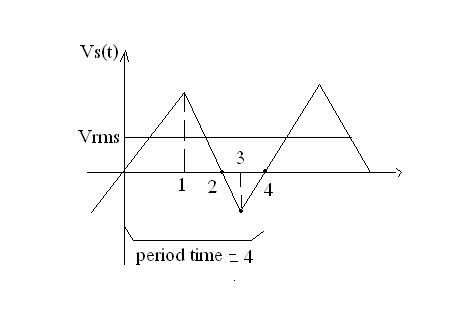# Root mean square question

calculate the RMS (root mean square) of this function.the period T=4
the formula is

$$V_{rms}=\sqrt{\frac{1}{T}\int_{0}^{T}V_r^2dt}$$
$$V_{rms}=\frac{1}{4}4\int_{0}^{T}(4t)^2dt}$$
$$V_rms=\sqrt{s}=\sqrt{\frac{16}{3}}$$
the solution says that they divide the graph into 4 traingles
and they sum their areas

but they dont use the integral?
why they say (4t)^2
from where the 4t comes from?

why they say that its the root of the area
why they dont divide by the period?

Last edited:

jambaugh
Gold Member
calculate the RMS (root mean square) of this function.
http://i50.tinypic.com/2iuq80x.jpg
the period T=4
the formula is

$$V_{rms}=\sqrt{\frac{1}{T}\int_{0}^{T}V_r^2dt}$$
$$V_{rms}=\frac{1}{4}4\int_{0}^{T}(4t)^2dt}$$
$$V_rms=\sqrt{s}=\sqrt{\frac{16}{3}}$$
the solution says that they divide the graph into 4 traingles
and they sum their areas

but they dont use the integral?
why they say (4t)^2
from where the 4t comes from?

why they say that its the root of the area
why they dont divide by the period?

Note you can include the picture inline by bracketing with IMG and /IMG like this:Your picture doesn't show the vertical scale. I assume from the answer you are getting that the peaks are 4 units above and below zero and so (though it doesn't look like it in the graph) all four triangles in question are the same dimensions 4x1.

Since you are squaring the amplitudes you'll note that each trangle contributes the same amount to the mean square (and thus RMS).
(They shouldn't say you add the areas but rather add the contributions = integrals of the square magnitudes. That may be what confused you.)

The first triangle is the area under the line V = 4t
(solve for line through the origin and through $$V(1)=4$$)
and thus they integrate the square of this to get:

$$C_1 = \int_{0}^{1}V(t)^2dt = \int_0^1 16t^2dt = \left. \frac{16t^3}{3}\right|_{t=0}^{t=1} = \frac{16}{3}$$

The $$C_1$$ stand for "contribution of 1". You get 4 such contributions from each triangle. That's the multiplicative 4. They also divide by 4 since that's the period.

$$(V_{rms})^2 = \frac{4_{(contributions)}}{4_{(period)}}\cdot \frac{16}{3}$$
so
$$V_{rms} = \sqrt{\frac{16}{3}}$$

Understand?

i dont understand what is the function of C1
why V(1)=4
?

after that i understood the integral of C1 and it result.
next you multiply by 4 because of 4 such contributions
and you divide by 4 because period=4

jambaugh
Gold Member
i dont understand what is the function of C1
why V(1)=4
?

Why is because it gave the same answer as you quoted. I assumed V(1) = 4 and V(3) = -4.
But we shouldn't be assuming this. The problem must have given this value, probably in the original graph.

That gives V(t) = 4t for the first unit of time which is what is being squared in the integrand.

If not then let V(1) = u = -V(3) and V(t) = ut for 0<t<1. Then your answer is:
$$V_{rms} = \sqrt{\frac{u^2}{3}}$$

thanks :)